Courses
Courses for Kids
Free study material
Free LIVE classes
More

# Octal NumbersLIVE
Join Vedantu’s FREE Mastercalss

## Addition and Subtraction of Octal Numbers: Introduction

The ‘Sum' of two integers is the place in the ‘sum' area where these two columns overlap,octal number addition follows the same rules as decimal or binary number addition. Octal addition is performed similarly to decimal addition.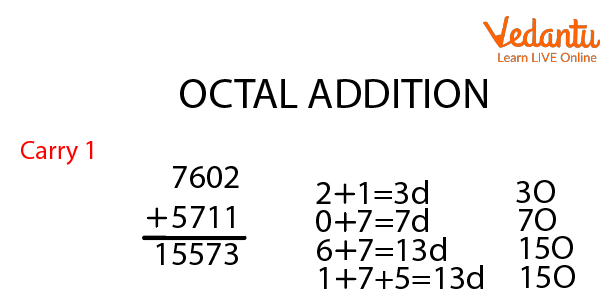## How Does the Octal Number System Work?

The Octal Number System is a number system having an 8 as its base and digits ranging from 0 to 7. The term octal refers to numerals with an eight as their base. Octal numbers have numerous uses and are widely used in computers and digital numbering systems. Octal numbers can be converted to binary numbers, decimal numbers, and hexadecimal numbers in the number system.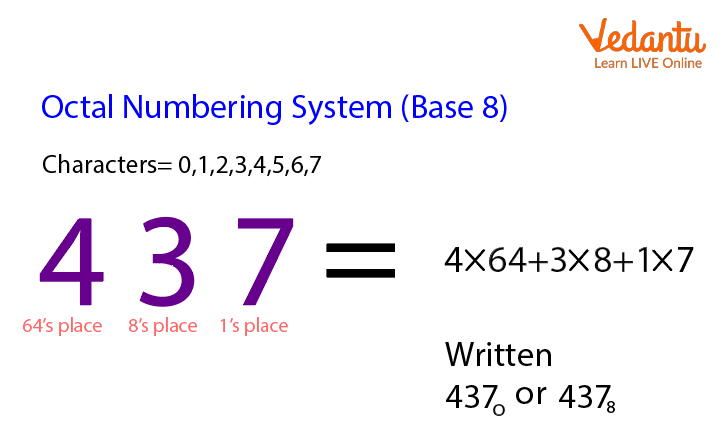Numbering System

## Octal Number Chart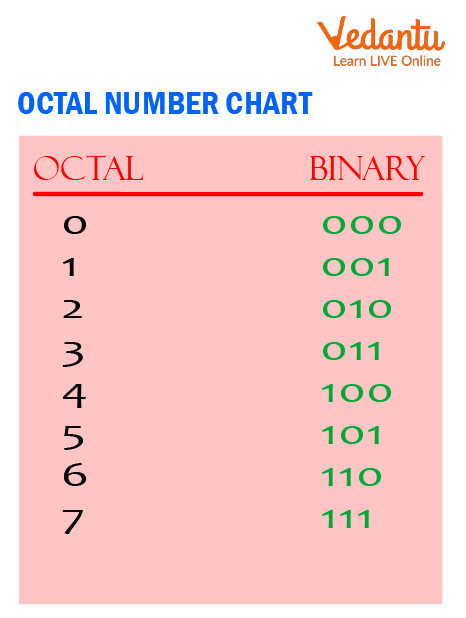Octal Numbers Chart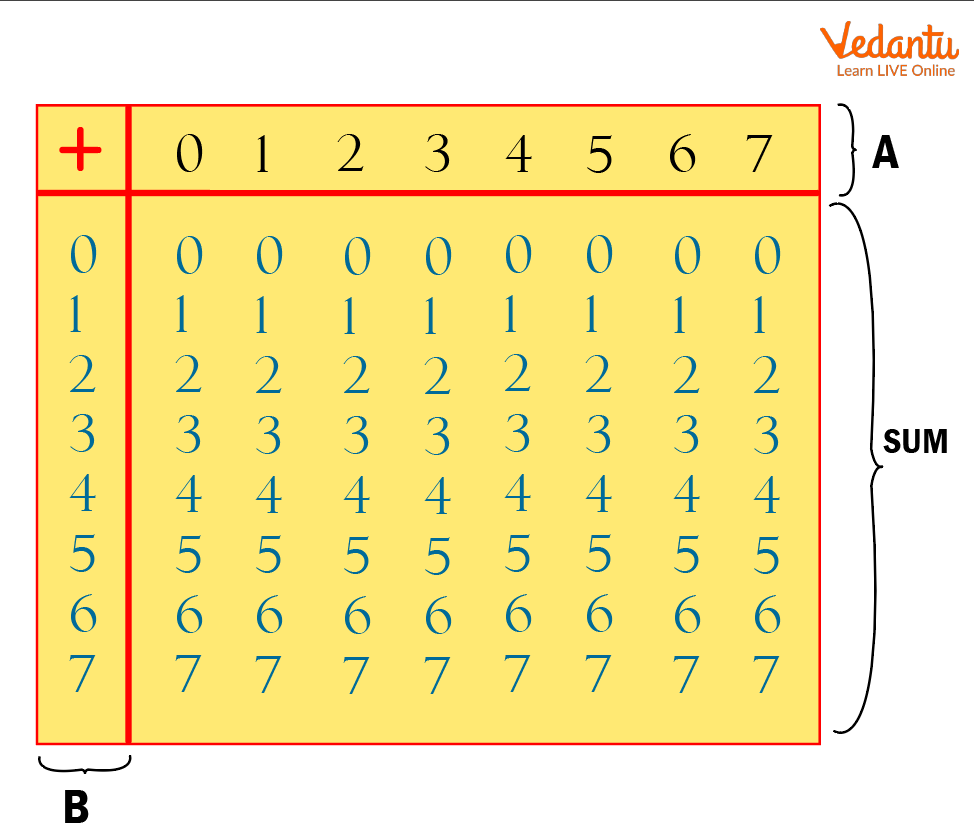68 + 58 = 138.

Simply follow the instructions in this example to use this table: Add 68 and 58 together. Locate 6 in the A column, then 5 in the B column. The ‘Sum' of two integers is the place in the ‘sum’ area where these two columns overlap.

## How To Convert Octal To Decimal Form?

Octal to Decimal conversion is similar to other number system conversions such as decimal to octal, octal to hexadecimal, octal to binary, and so on. When converting from octal to decimal, an octal number with the base of 8 must be converted to a decimal number with the base of 10. When we wish to know the number system equivalent of a number, we convert it from octal to decimal. There are four sorts of number systems: binary number systems, octal number systems, decimal number systems, and hexadecimal number systems. Each number system has its own set of base numbers that aid in determining the type of number. These base numbers are also useful in converting octal to decimal. The base number for octal numbers is 8 and for decimal numbers it is 10.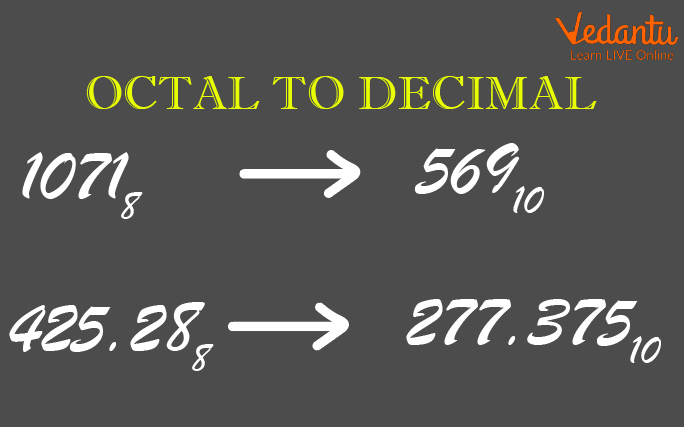Octal to Decimal Conversion

## Steps To Convert Octal To Decimal :

Here are the steps for converting an octal number to a decimal number:

• Step 1: Because an octal number can only include digits ranging from 0 to 7, we must first arrange the octal number with the power of 8.

• Step 2: We calculate the value of each octal number by evaluating all power of 8 values such as 80 is 1, 81 is 8, and so on.

• Step 3: Once we have the value, we multiply each number.

• Step 4: To acquire the decimal number, sum the product of all the numbers.

Consider the following example: convert (140) to 8 base, into a digit number

Step 1: Write 140 multiplied by the power of 8. Begin on the right-hand side.

1 × 82+ 4 × 81 + 0 × 80

Step 2: For each octal number, calculate the power of 8 values.

82 = 64, 81 = 8, 80 = 1

Step 3: Multiply each power of 8 by the corresponding number.

1 × 64 + 4 × 8 + 0 × 1 = 64 + 32 + 0

Step 4: Add the values together to get the decimal number.

64 + 32 + 0 = 96.

Therefore,( 140)= (96) to the base 10 .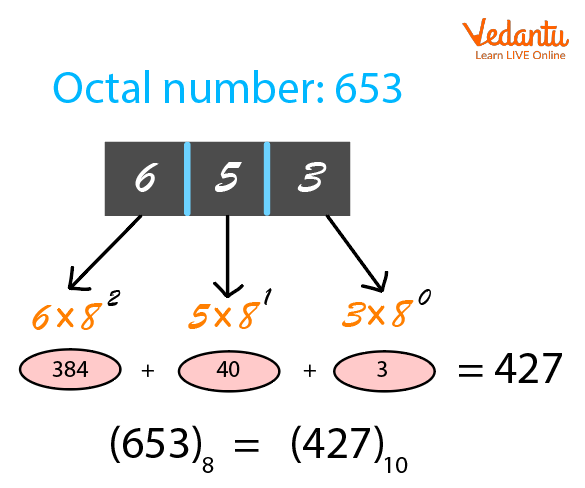Octal to Decimal Conversion

## Solved Questions

1. What is octal?

Ans: Employing a system of numerical notation with a base of 8 rather than 10 Choosing an octal number over a binary number saves digits. Octal was commonly employed in the early days of computers.

2. What is octal and binary?

Ans: Base 8 for octal numbers and base 2 for binary numerals We can translate the octal number to decimal, and then the decimal number to its binary equivalent.

3. What is the difference between octal, decimal and binary and hexadecimal?

Ans: In the number system, numbers are represented by their bases. Numbers have four bases: binary, octal, decimal, and hexadecimal. The base is binary if it is 2, octal if it is 8, decimal if it is 10, and hexadecimal if it is 16.

## Summary

The main benefit of adopting Octal numbers is that they have fewer digits than the decimal and hexadecimal number systems. As a result, there are fewer computations and fewer computational errors. It just requires three bits to represent each digit in binary and is simple to convert from octal to binary and vice versa. However, octal numbers are no longer commonly used, and, as previously stated, the hexadecimal numbering system has taken their place. One disadvantage of the octal number system is that computers can not understand octal numbers directly, thus they must first be transformed to binary numbers.

Last updated date: 22nd Sep 2023
Total views: 82.8k
Views today: 2.82k

## FAQs on Octal Numbers

1. How many 4 digits numbers can be formed in an octal number system?

In the octal numbering system, binary numbers are represented by a set of three digits (2 to the power 3= 8). The octal number system has eight numbers ranging from 0 to 7. They are as follows: 0, 1, 2, 3, 4, 5, 6, and 7.

2. Convert binary number (001100101101110)2  to the octal number

First write the 3-bit binary number for the mentioned binary number according to the table i.e 1, 4, 5, 0, 5, 6

Therefore, (001100101101110)2 = (14556)8

3. Convert (317)8 into Decimal.

The conversion from octal to decimal can be done in the following way: (317)8 = 3 × 82+ 1  × 81 + 7 × 80 = 207.

4. Convert the Binary Number (11001111)2 to Octal.

The three-bit group of binary numbers can be written as 011,001,111 because we have to add a zero before each number to complete the grouping in the form of three binary digits. Therefore, the octal numbers will be 3, 1, 7 i.e., (317)8

5. Convert to an octal number if the decimal is 560

If the number 560 is a decimal,

Then 560/8=70, with no remainder

70/8 equals 8, and the remaining is 6

The remainder is 0 since 8/8=1

And ⅛ =0 with 1 as the remainder.

As a result the octal number ranges from MSD to LSD,

I.e. (1060)8

As a result(560)10=(1060)8